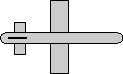# Maths - AxisAngle to Quaternion

## Prerequisites

Definition of terms:

## Equations

qx = ax * sin(angle/2)
qy = ay * sin(angle/2)
qz = az * sin(angle/2)
qw = cos(angle/2)

where:

• the axis is normalised so: ax*ax + ay*ay + az*az = 1
• the quaternion is also normalised so cos(angle/2)2 + ax*ax * sin(angle/2)2 + ay*ay * sin(angle/2)2+ az*az * sin(angle/2)2 = 1

## Code

Java code to do conversion:

```// assumes axis is already normalised
public void set(AxisAngle4d a1) {
double s = Math.sin(a1.angle/2);
x = a1.x * s;
y = a1.y * s;
z = a1.z * s;
w = Math.cos(a1.angle/2);
}```

## Derivation of Equations

This can be proved as follows:

from trig formula we get

cos(angle/2)2 + sin(angle/2)2 = 1

multiplying th sine part by 1 = ax*ax + ay*ay + az*az will have no effect so we can write:

cos(angle/2)2 + (ax*ax+ ay*ay + az*az) * sin(angle/2)2 = 1

expanding out gives:

cos(angle/2)2 + ax*ax * sin(angle/2)2 + ay*ay * sin(angle/2)2+ az*az * sin(angle/2)2 = 1

This shows that the quaternion is normalised since it is in the form:

qw2 + qx2 + qy2 +qz2 =1

and if we take the square root of each of these terms we get the parts of the quaternion:

qw = cos(angle/2)
qx = ax * sin(angle/2)
qy = ay * sin(angle/2)
qz = az * sin(angle/2)

That last part was not exactly a rigourous proof, but we can easily check that it is correct by checking rotations about each axis seperately as is done here.

## Example

 we take the 90 degree rotation from this:to this:As shown here the axis angle for this rotation is:

angle = 90 degrees
axis = 1,0,0

So using the above result:

cos(45 degrees) = 0.7071

sin(45 degrees) = 0.7071

qx= 0.7071

qy = 0

qz = 0

qw = 0.7071

this gives the quaternion (0.7071+ i 0.7071) which agrees with the result here

## Angle Calculator and Further examples

I have put a java applet here which allows the values to be entered and the converted values shown along with a graphical representation of the orientation.

Also further examples in 90 degree steps here